Your browser doesn't support the features required by impress.js, so you are presented with a simplified version of this presentation.

For the best experience please use the latest Chrome, Safari or Firefox browser.

# Multiple Linear Regression

• Multiple linear regression is a generalization to simple linear regression
• One Response v.s. multiple predictors
• $y=\beta_0+\beta_1x_1+\beta_2x_2+\cdots+\beta_px_p+\varepsilon$

# Scatterplot Matrix

• A convenient way to draw scatterplots for many variables
• Each cell is a scatterplot for the two corresponding variables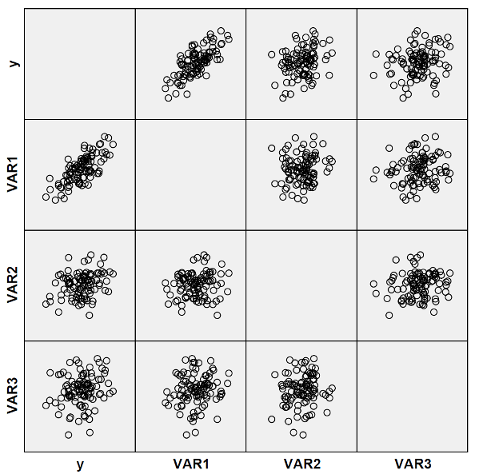# Fitting the Model

• Model summary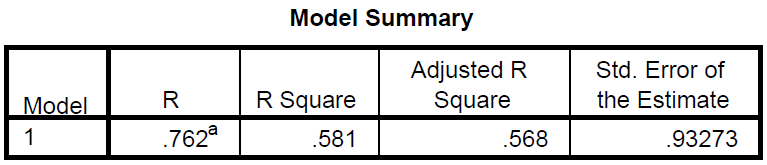• Coefficients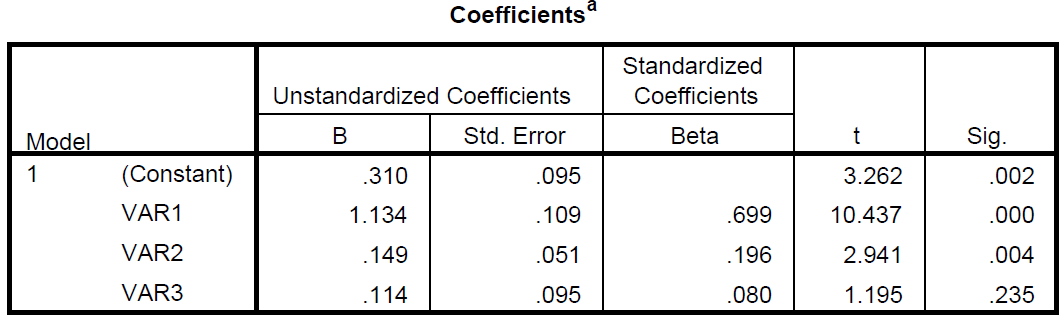# Fitting the Model

• ANOVA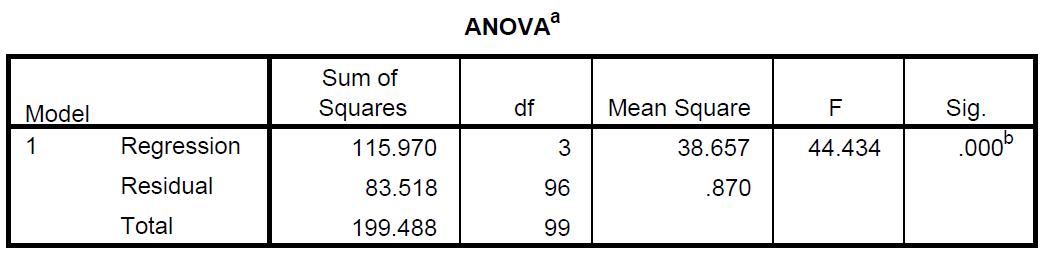# Refine the Model

• Drop insignificant variables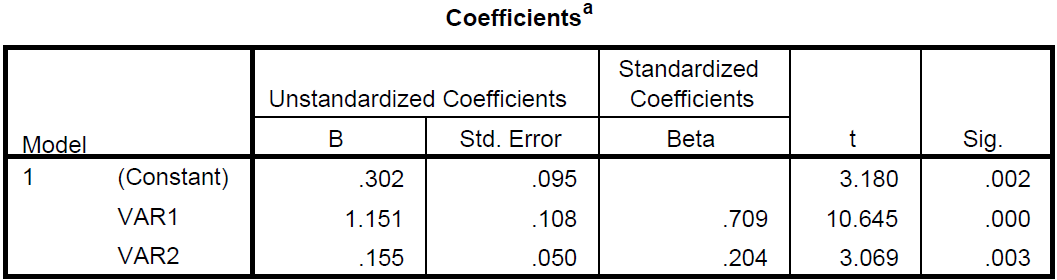• $R^2$ not decreasing much
• Before: 0.581; After: 0.575
• Std. error not increasing much
• Before: 0.93273; After: 0.93478

# Prediction and residual

• Using the regression equation to do prediction

$\hat{y}=0.302+1.151*Var1+0.155*Var2$

• Residual = True value - predicted value
• Look into original data to find the true value
• Use the predicted value above